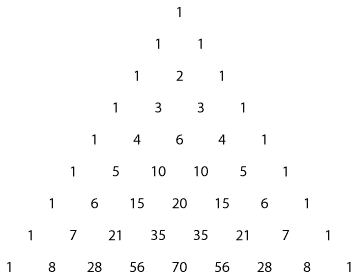## Content

### A look at Pascal's triangle

We begin by looking at the expansions of $$(1+x)^n$$ for $$n=0,1,2,3,4,5$$.

\begin{align*} (1+x)^0&=1\\ (1+x)^1&=1+x\\ (1+x)^2&=1+2x+x^2\\ (1+x)^3&=1+3x+3x^2+x^3\\ (1+x)^4&=1+4x+6x^2+4x^3+x^4\\ (1+x)^5&=1+5x+10x^2+10x^3+5x^4+x^5 \end{align*}

When the coefficients in the expansions of $$(1+x)^n$$ are arranged in a table, the result is known as Pascal's triangle.

Pascal's triangle
$$n$$ $$x^0$$ $$x^1$$ $$x^2$$ $$x^3$$ $$x^4$$ $$x^5$$ $$x^6$$ $$x^7$$ $$x^8$$
0 1
1 1 1
2 1 2 1
3 1 3 3 1
4 1 4 6 4 1
5 1 5 10 10 5 1
6 1 6 15 20 15 6 1
7 1 7 21 35 35 21 7 1
8 1 8 28 56 70 56 28 8 1

Pascal's triangle is often displayed in the following way. Some of the patterns of the triangle are more apparent in this form.Detailed description of diagram

By examining Pascal's triangle, we can make the following observations, which will be proved later in this module.

1. Each number is the sum of the two numbers diagonally above it (with the exception of the 1's).
2. Each row is symmetric (i.e., the same backwards as forwards).
3. The sum of the numbers in each row is a power of 2.
4. In any row, the sum of the first, third, fifth, $$\dots$$ numbers is equal to the sum of the second, fourth, sixth, $$\dots$$ numbers. (This is not a totally obvious result.)

We can use Pascal's triangle to help us expand expressions of the form $$(1+x)^n$$.

#### Example

Expand

1. $$(1+x)^6$$
2. $$(1-2x)^6$$.

#### Solution

1. The coefficients of $$(1+x)^6$$ are given in the sixth row of Pascal's triangle: $(1+x)^6 = 1 + 6x + 15x^2 + 20x^3 + 15x^4 + 6x^5 + x^6.$
2. The expansion of $$(1-2x)^6$$ can be obtained by replacing $$(-2x)$$ for $$x$$ in the expansion of $$(1+x)^6$$: \begin{align*} (1-2x)^6 &=1 + 6(-2x) + 15(-2x)^2 + 20(-2x)^3 + 15(-2x)^4 + 6(-2x)^5 + (-2x)^6\\ &=1 - 12x + 60x^2 - 160x^3 + 240x^4 - 192x^5 + 64x^6. \end{align*}

Next page - Content - Expansions and the notation